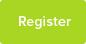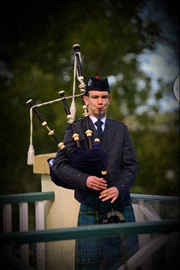# Quiver Mutations

Final year group investigation (Abstract and Sections 1, 2, 3 only) [format is a bit strange, but couldn`t upload original pdf version]

Date : 28/09/2016### Author Information

Subject : Maths

The aim of this report is to investigate a relatively new area of mathematics known as Quiver Mutations,first developed by S. Fomin and A. Zelevinsky in their theory of cluster algebras. Quivers are defined to be a directed graph made up of arrow and vertices. The condition for a quiver to be mutable is that it has no loops or 2-cycles. The definition of quiver mutation is given and some interesting properties that always hold when mutating quivers are observed. Two quivers are mutation equivalent if you can get from one to the other by finitely many mutations. The set of all utation equivalent quivers is called a mutation equivalence class. The formal definition of quiver isomorphism is given and what is meant by two quivers to have the same underlying graph. We then proceed by investigating several different types of quivers with the help of a Java applet. We look at each ones mutation equivalence class and state the interesting results we find. For example, the number of quivers in the mutation equivalence class of Ar generates a known sequence dependent on r. A cyclic quiver can be mutation equivalent to an acyclic one, but this is not always true and an example is given. We explain

how quivers are in one-one correspondence with skew-symmetric matrices and illustrate how to mutate a quiver in matrix form. Our investigation goes further as we attempt to solve a prize winning problem found on the web. A quiver is given and we need to find an acyclic mutation equivalent quiver to it in less than eight mutations. We decide to tackle it by creating our own function in R that can mutate a quiver in matrix form and check for cycles. Unfortunately we did not succeed in solving this problem with the tools we had at hand.

Introduction

Quiver mutation is a concept that was developed by Sergey Fomin and Andrei Zelevinsky and has many applications in both mathematics and physics. It made its first appearance in mathematics in the definition of cluster algebra discovered by Fomin-Zelevinsky in 2000 . Our aim in this project is to investigate this relatively new topic that we find greatly stimulating. There are very limited sources available both on the web and in books, but as a result of this, our project is completely independent from other mathematicians` work.

Throughout the investigation we perform quiver mutation in three different ways: mutating by hand, with the help of online computational software, and in matrix form. We seek to discover what properties are preserved in quiver mutation and which are not.

Quivers

A quiver is, in common usage, a container for holding arrows. In mathematics, a quiver is also a collection of arrows but more precisely it is a directed graph where loops and multiple arrows between two vertices are permitted. Quivers are commonly used in representation theory to reduce the complexity of some problems of abstract algebra to more combinatorial problems . The formal definition is as follows.

Definition. Quiver We define Q = (Q0Q1) to be a quiver, where Q0 is the vertex set and Q1 is the arrow set of Q (i.e. Q1 is a set of ordered pairs of elements of Q0, so that (a b) corresponds to an arrow from a to b).

We allow weights to be assigned to arrows, that is we may associate an arrow with an integer value. When there are multiple arrows between two vertices, it is often more convenient to replace these arrows with one arrow and assign it a weight equal to the sum of the weights of the arrows between them (the weight of arrows with opposite direction needs to be subtracted).

A vertex of a quiver can be of three types:

1. A source: only has arrows going out.

2. A sink: only has arrows going in.

3. Neither a source nor a sink: has both arrows going in and arrows going out.

In particular, we are interested in the mutation of quivers, however not all quivers can be mutated. We need to exclude those quivers that have loops, that is to say arrows whose source and target are the same, and 2-cycles, pairs of distinct arrows such that the source of one is the target of the other and vice versa. Mutating a quiver generates a new quiver by altering the arrows at a given vertex. The number of vertices in the quiver stays the same, while arrows can be created, inverted and destroyed. Quiver mutation

Quiver mutation was first introduced by S. Fomin and A. Zelevinsky in their theory of cluster algebras  and is defined in the following way.

Let Q be a mutable quiver. The mutation of Q at the vertex k is denoted _k(Q) is and is found by proceeding as follows:

1. Reverse all arrows incident with k.

2. For any pair of arrows in Q from i to k and k to j with weight r and s respectively, add a new arrow from i to j of weight equal rs.

3. If an arrow is already present from i to j, add its weight to the weight of the new arrow. If an arrow from j to i is present subtract its weight from the weight of the new arrow (if this is negative reverse the arrow).

A simple observation can immediately be made, that is: mutating a quiver at a source turns it into a sink and vice-versa. This follows from the fact that sources and sinks have no paths through them so only the first step of quiver mutation is necessary. This simply reverses all arrows incident with the source or sink, hence a source will become a sink and a sink will become a source.

An important feature of quiver mutation is that it has the property of being involutive. That is to say, if you mutate a quiver at the same vertex twice you return to the original quiver (µk(µk(Q)) = Q).

Proof. Let Q be a mutable quiver. Mutate Q at a vertex k and denote this by µk(Q). Now if k is a source/sink then we have already seen how these 2 types of vertex are interchanged via quiver mutation and so the property of involution is immediately apparent in this case. Therefore, let us assume that k is neither a source nor a sink and so there exists at least one path through k. Also, since Q is a mutable quiver we know that 2-cycles are not permitted.

Let us examine the effects of mutation on an arbitrary arrow path from, say, vertex i to k to j. Denote the weights of (i k) (k j) by s and t respectively, with s t ∈ N. Then we first reverse them both so that we now have a path from j to k to i. Next we add a new arrow of weight st going from i to j and by convention we may combine this with any arrow that already exists between i and j, so we now have an arrow from i to j of weight r + st where r ∈ Z is the weight of the existing arrow between i and j. Note that r = 0 is the case where no prior arrows existed and r < 0 is the case where the prior arrow was directed from j to i. r + st need not be positive but by allowing for it to have a negative weighting we may assume it is directed from i to j.

Now if we mutate at k again we first reverse all the arrows incident with k so that (i k) and (k j) are going in their original directions again. We now add an arrow of weight st from j to i. The resultant arrow from i to j will have weight r + -

This resource was uploaded by: Joshua